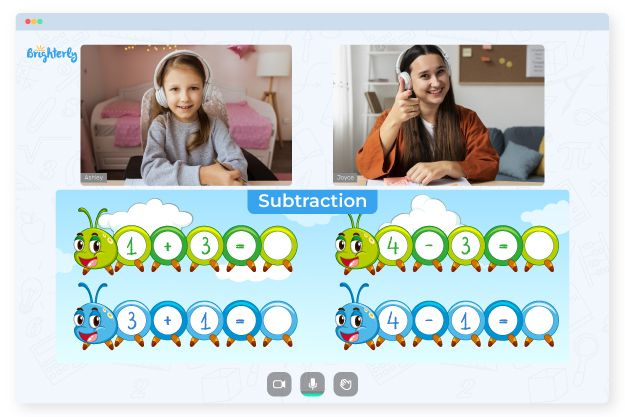# Single Digit Subtraction Worksheets

Subtraction single digit worksheets teach one of the basic arithmetic operations in mathematics — subtraction. These tools develop students’ conceptual understanding of subtraction, prepare them for learning division, and build their interest in math overall. So, if you are looking for proof that these worksheets are valuable for your grade school child, this article will help you greatly.

## About Single Digit Subtraction Worksheets

One digit subtraction worksheets include exercises that develop a students’ basic arithmetic skills. These math worksheets use a variety of interactive and simulated activities to help engage kids. Children can learn effectively by solving many fun visual tasks in these worksheets. The effective learning models give them confidence to face any problem that has to do with single-digit addition.

Math for Kids

Is Your Child Struggling With Math?
1:1 Online Math TutoringThe one digit subtraction worksheet contains tasks like sorting odd numbers, finding missing numbers, and more. You can download a single digit subtraction worksheet as a free PDF on platforms like Brighterly. And don’t worry about the tasks being too difficult for your child. Most times, the problems in these worksheets could appear in the order from the simplest  to more challenging ones, but there are precise, clear, and straightforward instructions kids can follow to improve.### Single Digit Subtraction Worksheets PDF

Subtraction Single Digit Worksheets### Single Digit Subtraction Worksheets PDF

Single Digit Subtraction Worksheet### Single Digit Subtraction Worksheets PDF

One Digit Subtraction Worksheets### Single Digit Subtraction Worksheets PDF

One Digit Subtraction Worksheet

### More Worksheets

Trouble with Subtraction?• Does your child find the concept of subtraction confusing?
• Start learning with an online tutor.

Is your child struggling with the basics of subtraction? Try studying with an online tutor.